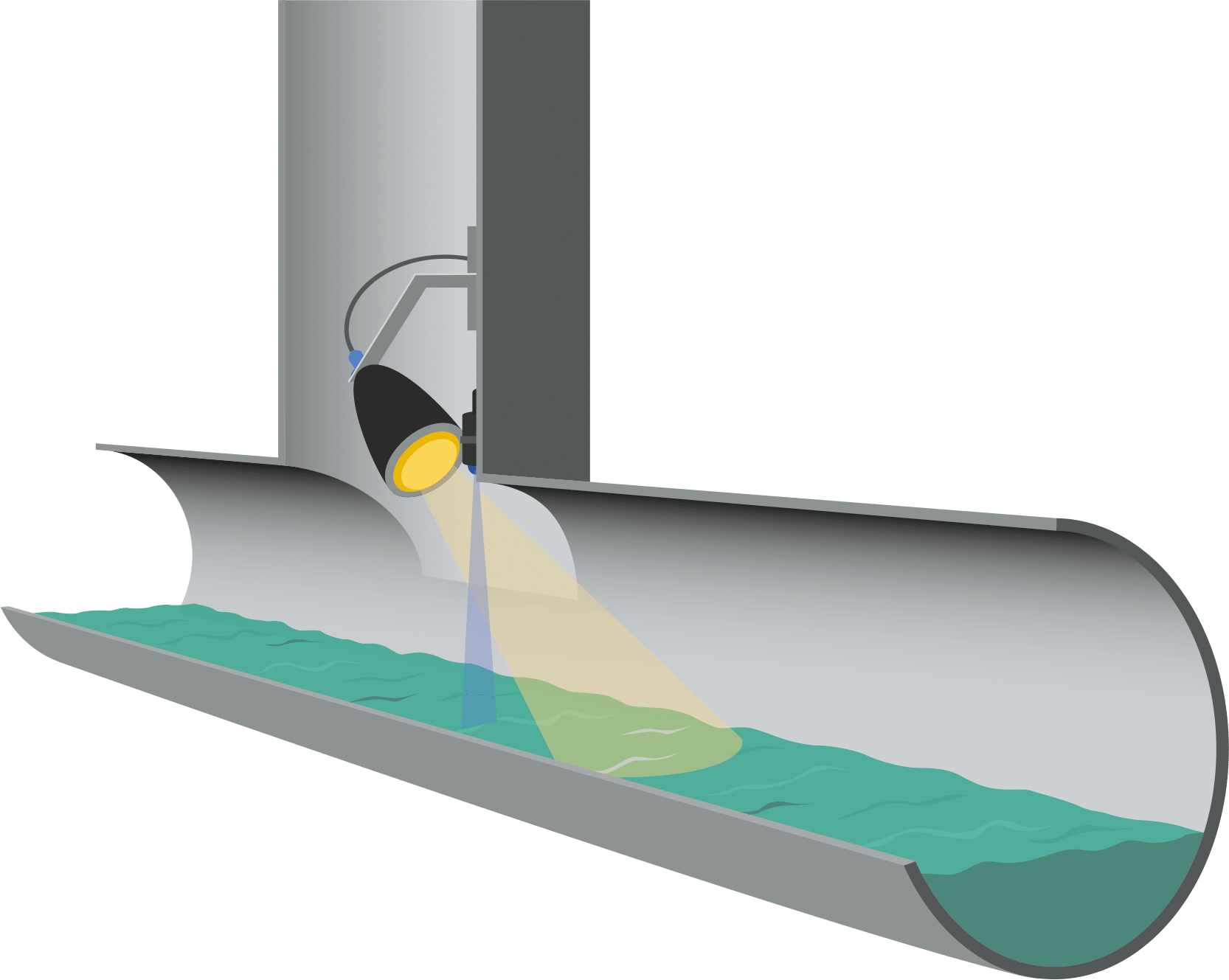## How To Calculate Sonic Velocity

In this post I want to share how to calculate sonic velocity. Before we jump into the equation, let’s review about sonic velocity briefly.

Sonic velocity or critical velocity is the maximum velocity that can be attained by a compressible fluid. We usually use this parameter in sizing pipe, control valve, and flare.

Sonic velocity is a function the following parameters:

• Ratio of specific heats in the gas (k or Cp/Cv)
• Temperature
• Molecular weight of the gas

The molecular weight is expressed in absolute temperature, therefore we will use temperature unit of oR or oK. Read More